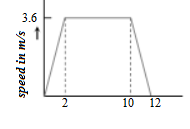Checkout NEET 2022 Question Paper Analysis : Checkout NEET 2022 Question Paper Analysis :

# Third law of motion MCQs For NEET

Newton’s third law of motion states that for every action there is an equal and opposite reaction. This statement means that for every interaction, there is a pair of forces acting on the two interacting objects. The size of the forces on the first object equals the size of the force on the second object. When we jump, our legs exert a force on the ground, and the ground exerts an equal and opposite force that propels us into the air.

1. Walking is possible on the account of

1. Newton’s First Law of Motion
2. Newton’s Second Law of Motion
3. Newton’s Third Law of Motion
4. Newton’s Law of Gravitation

Answer: (c)Newton’s Third Law of Motion

2. When we jump out of the boat standing in still water, the boat moves

1. Forward
2. Backwards
3. Sideways
4. It doesn’t move

3. A man is at rest on perfectly smooth ice in the middle of a pond. Using the principle of which law will get him to the shore?

1. Newton’s First Law
2. Newton’s Second Law
3. Newton’s Third Law
4. All the above three laws

4. Recoiling of a cannon after firing a shot is an example of

1. Newton’s First Law
2. Newton’s Second Law
3. Newton’s Third Law
4. Newton’s Law of Gravitation

5. A body floats in a beaker that contains liquid. If the whole system as shown in the figure falls freely under gravity, then the upthrust on the body due to the liquid is

1. Equal to the weight of the displaced liquid
2. Equal to the weight of the body in the air
3. Zero
4. None of the above

6. The gun recoils after firing a bullet due to

1. Newton’sThird Law of Motion
2. The backward thrust of gases
3. Newton’s First Law of Motion
4. Conservation of Energy

Answer: (a) Newton’s Third Law of Motion

7. A lift carrying a passenger is going up and the total mass of passenger and the lift is 1500 kg. The variation in the speed of the lift is shown in the graph given below. What is the tension in the rope pulling the lift at t = 11 sec?1. 14700 N
2. 17400 N
3. 12500 N
4. 12000 N

8. In the above question, how high does the lift take the passenger?

1. 36 meters
2. 3.6 meters
3. 44 meters
4. 4.4 meters

9. Why won’t the man attempting to pull himself by tugging on his hair not succeed?

1. The frictional force in play while gripping is small.
2. The exerted force is small.
3. The force applied is internal to the system.
4. Newton’s law of inertia is not applicable to living beings.

Answer: (c) The force applied is internal to the system.

10. The horse pulling the wagon is able to move forward because of

1. the force exerted by the horse on the ground
2. the force exerted by the ground on the horse
3. the force exerted by the wagon on the horse
4. the force exerted by the horse on the wagon

Answer: (b) the force exerted by the ground on the horse

Stay Tuned to BYJU’S for more updates on NEET.

Related articles on NEET Physics: